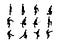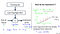# Introduction

If we believe the scientists, initially, there was a magical Big Bang giving birth to time and space. Millions and billions of years later, in an obscure part of the universe, a small mass called Earth with the perfect environment for the evolution of living organisms was formed. Then following the laws of nature, organisms from bacteria to humans evolved. Fighting, surviving, communicating, and innovating, humans dominated the Earth. With some luck, our ancestors found their partners and passed on their genes to the next generation. Somewhere along the way, your parents met and gave birth to you on that…

# Introduction

Game of Life is a fascinating thought game/board game/computer algorithm with simple rules having profound consequences. It was invented in 1970 by the Cambridge mathematician John Conway and popularized by a series of articles in "Scientific American" by Martin Gardner. After 50 years of its original publication, thousands are still not just playing it but conducting actual research and asking philosophical questions.

# The Rules

Conway’s game of life starts with an infinite grid of squares. Each square has 8 touching neighbors. Each cell can be in two states: dead or alive. Initially, all the cells are dead cells. …

# Numpy Vectorization Speed Test with Random Walk ExampleMonty Python’s Silly Walk Step by Step Tutorial
`import numpy as npimport random`

# Random Walk

A random walk is a path that has no clear direction but is determined by a series of random decisions, each of which is left entirely to chance. For example, a pollen grain floating on a drop of water moves across the surface of the water because it’s constantly pushed around by water molecules. Molecular motion in a water drop is random, so the path a pollen grain traces on the surface is a random walk. 

The random walk hypothesis is a financial theory stating that stock market prices evolve according to a…

# pandas: The Python Data Analysis Library

• This Python package is designed on the basis of the NumPy library.
• Another fundamental choice was to design ad hoc data structures for data analysis.
• These data structures are designed to work with relational data or labeled data, thus allowing you to manage data with features similar to those designed for SQL relational databases and Excel spreadsheets.
• First of all, we need to import pandas and numpy.
`import pandas as pdimport numpy as np`

## Introduction to pandas Data Structures

The heart of pandas are the two primary data structures,

• Series (data structure designed to accommodate a sequence of one-dimensional data)
• Dataframes (complex data structured…

# Ndarray: The Heart of the Library

• Numpy library is based on one main object ndarray (N-dimensional array)
• Ndarray is multidimensional homogeneous (all items are the same type and same size) array.
• the shape defines the tuple of integers giving the size of the array along each dimension.
• dimensions are defined as axes
• rank is the number of axes
• size gives array length
`>> import numpy as np>> a = np.array([        1,2,3    ])>> b = np.array([        [1,2,3],        [2,3,4]    ])>> c = np.array([        [[1,2,3],        [4,5,6],        [7,8,9]],        [[10,11,12],        [13,14,15],        [16,17,18]]    ])>> print(f'a.shape {a.shape} \nb.shape {b.shape} \nc.shape {c.shape}')a.shape (3,) b.shape (2, 3) c.shape …`

# Linear Regression (Univariate Linear Regression)

Linear Regression is one of the strongest tools available in statistics and machine learning and can be used to predict some value (Y) given a set of features (X).

To understand the basics, let’s take a common example of predicting house prices in Boston. We are given several demographic and geographical attributes but let’s take a simple univariate linear regression predicting the price of a house on a single feature (size of the house).Univariate linear regression

We are given a training set, we train it using a learning algorithm to create a hypothesis. Given, the size of the house (x), hypothesis (h(x))…

# What is Machine Learning?

According to Arthur Samuel (1959),

It is a field of study that gives computer ability to learn without being explicitly programmed.

But, how is a computer supposed to learn? Tom Mitchell says that

A computer program is said to learn from experience E with respect to task T and some performance measure P, if its performance on T, as measured by P improves with experience E.

For example: Playing Checkers (Btw Arthur Samuel’s Checkers playing program was among the world’s first successful self-learning programs.) Here,

`E = the experience of playing many games of checkersT = the task of playing…`## Subash Basnet

I am a Computer Engineer graduated from Kathmandu University, Nepal. The existence is a program, we are here to add our part of code. Website: sbasnet.com.np